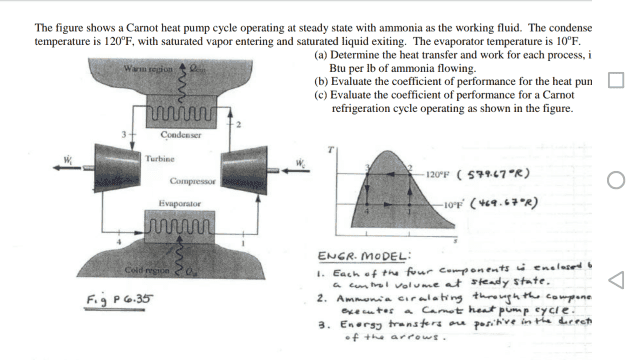# Conceptual thermodynamics problem about ammonia executing a Carnot cycle

• Andrew1234

#### Andrew1234

Homework Statement
Explain why the work done in process 2-3 is zero.
Relevant Equations
ΔH = ΔQ-ΔWIs there a mathematical explanation for why the work done in the condenser (in process 2 to 3) is zero? I am aware that ammonia does not expand or compress in the condenser, only changes phase, but without knowing that the process takes place in a condenser and only considering the graph, according to which temperature is constant for process 2-3, how can it be seen that no work is done in this process?

In process 2-3 the temperature and pressure are both constant. Because the cycle is reversible, Q = ∫Tds.

Substituting this in the energy balance, -m'(Δh)+∫Tds = ΔW. Because temperature is constant, ΔW = T(Δs)-m'(Δh).

W = TΔs - ΔH = T(sf-sg) + m(hg - hf)

It is not clear to me why this quantity is zero for process 2-3.

#### Attachments

Last edited:

Homework Statement:: Explain why the work done in process 2-3 is zero.
Relevant Equations:: ΔH = ΔQ-ΔW

View attachment 259982

Is there a mathematical explanation for why the work done in the condenser (in process 2 to 3) is zero? I am aware that ammonia does not expand or compress in the condenser, only changes phase, but without knowing that the process takes place in a condenser and only considering the graph, according to which temperature is constant for process 2-3, how can it be seen that no work is done in this process?

In process 2-3 the temperature and pressure are both constant. Because the cycle is reversible, Q = ∫Tds.

Substituting this in the energy balance, -m'(Δh)+∫Tds = ΔW. Because temperature is constant, ΔW = T(Δs)-m'(Δh).

W = TΔs - ΔH = T(sf-sg) + m(hg - hf)

It is not clear to me why this quantity is zero for process 2-3.
There is no way to know unless, as in the case of a condenser, the device has no shaft that does shaft work. In other words, you need to know something about how your device works.

Thank you for clarifying this concept

Both processes, 2 to 3 and 4 to 1, have only transfer of heat.
The value of delta work in the equation ΔH = ΔQ-ΔW is always zero for these processes.
In real systems, there are sub-cooling and overheating portions besides pure change of phase in these processes.